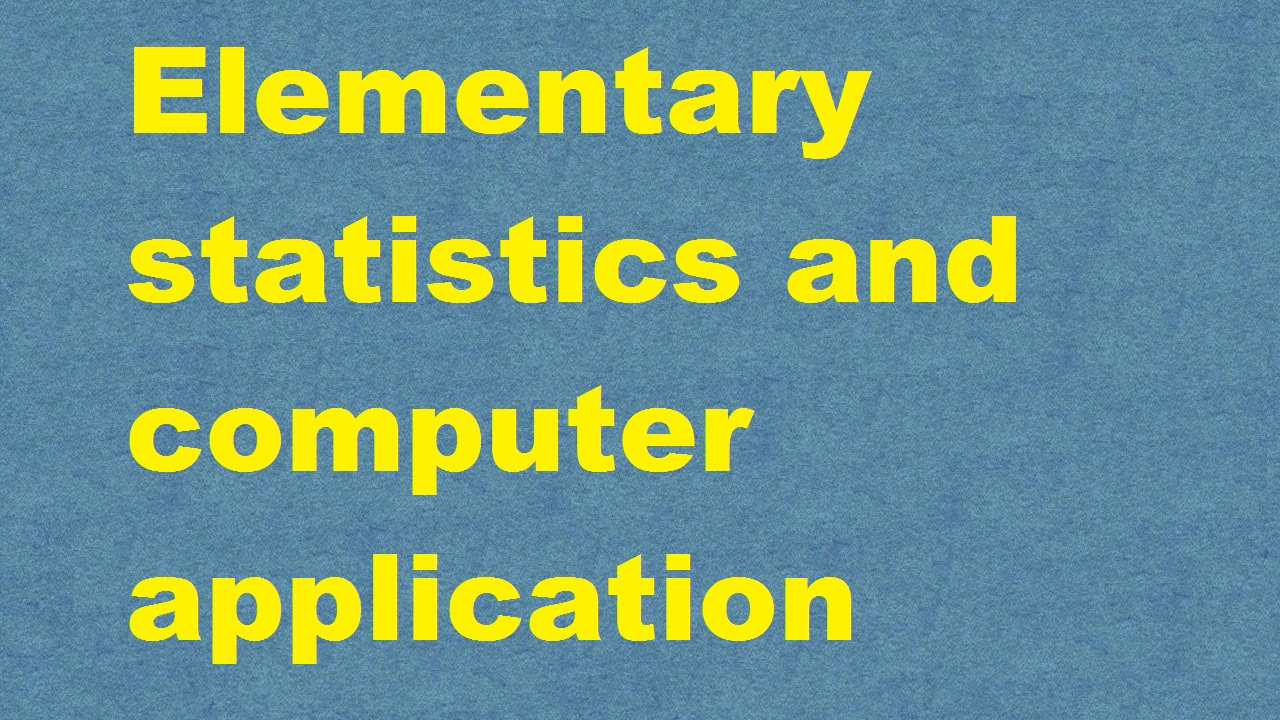# Elementary statistics and computer application ICAR Ecourse Free PDF Book Download e krishi shikshaElementary statistics and computer application ICAR Ecourse Free PDF Book Download e krishi shiksha

Elementary statistics and computer application ICAR Ecourse PDF Book was developed by ICAR e Krishi Shiksha and TNAU. You Can easily  Download it from our website IARI Toppers by clicking on the below given link.We have provided the latest version of Elementary statistics and computer application ICAR Ecourse PDF Book but some mistakes can be found. If you found  any mistake,  error in this PDF book than feel free to comment below.

### Course Contentof Elementary statistics and computer applicationICAR Ecourse Free PDF Book Download e krishi shiksha:

Theory
Basic concepts: Variable statistics, types and sources of data, classification and tabulation of data, construction of frequency distribution, tables, graphic representation of data, simple, multiple component and percentage, bar diagram, pie diagramhistogramfrequency polygonand frequency curve average and measures of location, mean, mode, median, geometric mean, harmonic mean, percentiles and quadrilles, for raw and grouped data. Dispersion: Rangestandard deviationvariance, coefficient of variation for raw and grouped dataProbabilityBasicconcept, additive and multiplicative laws. Theoretical distributions, binominal, poison and normal distributions, samplingbasic conceptssampling vs. complete enumeration parameter and statistic, sampling methods, simple random sampling and stratified random sampling. Tests of Significance: Basic concepts, tests for equality of means, and independent and paired t-tests, chi-square test for application of attributes and test for goodness of fit of mendalian ratios. CorrelationScatter diagramcorrelation co-efficient and its properties, regression, fitting of simple linear regression, test of significance of correlation and regression coefficient. Experimental Designs: Basic concepts, completely randomized design, randomized block design, latin square designs, factorial experiments, basic conceptsanalysis of factorial experiments up to 3 factors – split plot design, strip plot designlong term experiments, plot size, guard rows. Computer applicationIntroduction to computers and personal computers, basic concepts, operating system, DOS and Windows 95, introduction to programming languages, BASIC language, concepts, basic and programming techniques, MS Office, Win Word, Excel, Power Point, introduction to Multi-Media and its applicationVISUAL BASIC-concepts, basic and programming techniques, introduction to Internet.Practical
Construction of frequency distribution table and its graphical representation,histogramfrequency polygonfrequency curve, bar chart, simple, multiple, component and percentage bar charts, pie chart, mean, mode for row and grouped datapercentiles, quadrille, and medianfor row and grouped data, coefficient of variation, ‘t’ test for independent, will equal and unequal variants, paired ‘t’ test, chi-square test for contingency tables and theoretical ratios, correlation and linear regression. Studies on computer components – BASIC language, VISUAL BASIC, programming techniques, MS Office, Excel, Power Point.

### Watch Video Lecture of Elementary statistics and computer application ICAR Ecourse Free PDF Book Download:

You can also watch the online video lecture of Elementary statistics and computer application ecourse which will be more helpful to easily understand the lecture.

Disclaimer - The following video is the sole property of their respective owner / you tube channel and they owns all the ownership rights. We have embedded it here under fair uses for educational purposes only.
No Video Available

Hope you enjoyed our video lecture which covers the following topics:

Elementary statistics and computer application ICAR Ecourse Free PDF Book Download e krishi shiksha